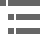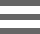## CCD Resolution for Optical MicroscopyThe ultimate resolution of a CCD is a function of the number of photodiodes and their size relative to the image projected onto the chip's surface by the microscope optics. When attempting to match microscope optical resolution to a specific digital camera, use this calculator to determine the necessary pixel density to adequately capture all of the data from the microscope.

To operate the tutorial, first select an optical aberration correction factor for the objective of interest using the Objective pull-down menu. Next, choose a magnification/numerical aperture combination from the Magnification pull-down menu. After these selections are made, the pixel calculator will automatically perform the necessary calculations to arrive at a required number of pixels for the CCD camera. This value will be displayed in the pink data box, labeled Minimum Pixels Required (millions) at the bottom of the tutorial window. Intermediate values of other important parameters employed in the calculation are displayed in the blue-gray data boxes located between the pull-down menus and the pink data box.

Adequate resolution of a specimen imaged with the optical elements of a microscope can only be achieved if at least two samples are made for each resolvable unit, although many investigators prefer three samples per resolvable unit to ensure sufficient sampling. In diffraction limited optical instruments, such as the microscope, the Abbe limit of optical resolution at an average wavelength of 550 nanometers is 0.22 microns when using an objective lens having a numerical aperture of 1.4. In this case, a sensor size of 11 x 11 microns would be just large enough to allow the optical and electronic resolution to be matched, with a 7 x 7 micron sensor size preferred. Although small sensors in a CCD improve the spatial resolution, they also limit the dynamic range of the device.

The following calculations, based on an average illumination wavelength of 580 nanometers, were utilized to fabricate this interactive tutorial. The microscope objective is assumed to have a 40x magnification factor at a numerical aperture value of 0.95 (plan apochromatic high-dry objective).

In a system where the numerical aperture of the objective and condenser are matched and equal to a value of 0.95, and using a value of 580 nanometers (0.58 microns) for the average wavelength, the solution becomes:

The image size projected onto the surface of the CCD detector equals the optical resolution multiplied by the objective magnification:

The objective used in these calculations is a 40x plan apochromat having a numerical aperture of 0.95. Thus, the image size projected onto the CCD surface is:

To convert the image size projected onto the CCD surface (0.01221 millimeters) into line pairs per millimeter, we take the reciprocal of the projected size:

Line pairs per millimeter can be converted into equivalent TV lines by multiplying this quantity by the vertical CCD dimension (in this case, 6.4 millimeters) times a factor of 2:

The CCD maximum resolution can now be calculated by multiplying the number of TV lines by the CCD aspect ratio (5/4 or 1.25 for this example).

Finally, the minimum number of pixels required to match the optical resolution of the microscope can be calculated by multiplying the number of TV lines times the CCD maximum resolution.

From the series of calculations performed above, for a 40x plan apochromatic objective operating with a numerical aperture of 0.95 and an illumination wavelength of 580 nanometers, the number of pixels necessary to acquire maximum optical data from the microscope is 1.4 million. This value falls into line with the resolution capabilities of many digital cameras currently offered by microscope manufacturers or as aftermarket add-ons.

### Contributing Authors

Brian O. Flynn and Michael W. Davidson - National High Magnetic Field Laboratory, 1800 East Paul Dirac Dr., The Florida State University, Tallahassee, Florida, 32310.

# Microscopy U - The source for microscopy education•••